KING FAHD UNIVERSITY OF PETROLUEM AND MINERALS

Faculty of Sciences   Prep Year math program

Math 001 - Term 022

Part I: ( Multiple Choice Questions)

1) The y-intercept(s) of the equation :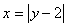is :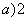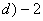2) The radius of the circle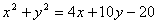is :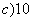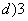3) Which of the following defines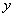as a function  of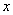: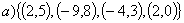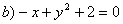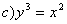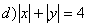4) If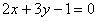and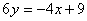are two straight lines, then which one of the

following is true :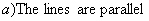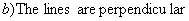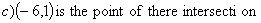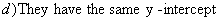5) The equation of the line passing throughis :b)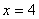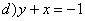6) The graph of the equation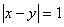is :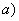two parallel lines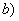two perpendicular linescircle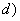only two points

7) If the point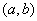lies in Quadrant II , then the point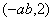lies in

8) Which one of the following is NOT TRUE :

a) The range of the greatest integer function is the set of all integers.

b)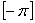= -3 .

9) If, then the range of the function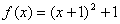is equal to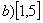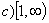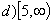10) If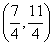is the midpoint of the line segment with end points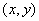and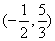,

then the value ofequal toPart II : Written Part

Question1: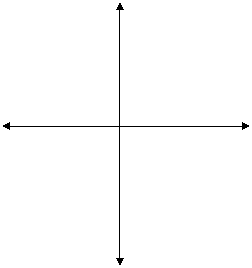Given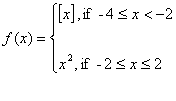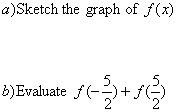Question2:

Given the circle :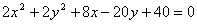a) Write this circle in the standard form.

b) Find the center and radius.

Question3:  Find the domain of the following functions

a)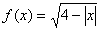b)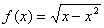Question4:

a) Find the equation of  a circle that  has diameter with endpointsand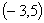.

b) Find the equation of a circle that has centerand tangent to the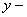axis.

Question5:

a) If the two lines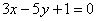and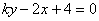are perpendicular, find k.

b) Find the-intercept of the line through the points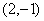and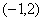.

Question10 :

Given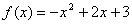.

a) Find the vertex .

b) Find the equation of the axis of symmetry .

c) Find the range of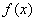d) sketch the graph of. ( Show the axis of symmetry ,-intercept(s),and-intercept(s) ).

e) Determine the interval in whichis increasing .

f) Determine the interval in whichis decreasing .

Question11:

Given the two points A(-2,3)  and B(-1,-5).

a) Find the equation of the line passing through the points A and B.

b) Find the distance between points A and B.

c) Find the midpoint of the line segment joining the points A and B.